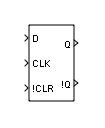# D Flip-Flop

Model a positive-edge-triggered enabled D flip-flop

• Library:
• Simulink Extras / Flip Flops

•## Description

The D Flip-Flop block models a positive-edge-triggered enabled D flip-flop. The D Flip-Flop block has three inputs:

• D — data input

• CLK — clock signal

• !CLR — enable input signal

On the positive (rising) edge of the clock signal, if the block is enabled (!CLR is greater than zero), the output Q is the same as the input D. The truth table for the D Flip-Flop block follows.

Note

The D Flip-Flop block treats a nonzero input as true (`1`).

DQ!Q
110
001

If the block is not enabled on the rising edge of the clock signal, Q is reset to zero. When the clock signal is not rising, the block remains in the previous state.

### Logic Signals as Boolean or Double Data Types

The Implement logic signals as boolean data (vs. double) configuration parameter setting affects the input and output data types of the D Flip-Flop block because the D Flip-Flop is a masked subsystem that uses the Logical Operator block. The Logical Operator block in the masked subsystem has the following parameter settings:

Block Parameter Setting
Output data type`Inherit: Logical`
Require all inputs and output to have the same data type`On`

For more information about the Implement logic signals as boolean data (vs. double) configuration parameter, see Implement logic signals as Boolean data (vs. double).

## Ports

### Input

expand all

Data input signal, specified as a scalar, vector, or matrix.

#### Dependencies

The data types that the D Flip-Flop block accepts for the input D depend on the setting of the Implement logic signals as boolean data (vs. double) model configuration parameter. If this parameter is on, D must have data type `boolean`; if this parameter is off, D can have data type `boolean` or `double`.

Data Types: `double` | `Boolean`

Clock signal, specified as a scalar, vector, or matrix.

Data Types: `single` | `double` | `int8` | `int16` | `int32` | `uint8` | `uint16` | `uint32` | `Boolean` | `fixed point` | `enumerated` | `bus`

Enable input signal, specified as a scalar, vector, or matrix.

Data Types: `single` | `double` | `int8` | `int16` | `int32` | `uint8` | `uint16` | `uint32` | `Boolean` | `fixed point` | `enumerated` | `bus`

### Output

expand all

Output signal Q, with the same dimensions and data type as the input D.

Data Types: `double` | `Boolean`

Output signal !Q, with the same dimensions and data type as the input D.

Data Types: `double` | `Boolean`

## Version History

Introduced in R2008b